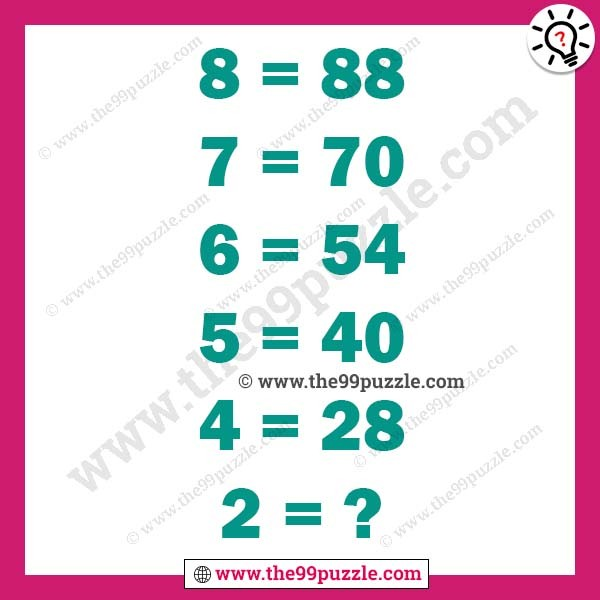# Maths logic tricky puzzle with answer – Puzz237

Now trying to solve this maths logic tricky puzzle. Puzzles help you to gain logical skills and quick solving. Puzzle lovers like to solve various types of brain teasers puzzles. Practice more puzzles that you make more genius and smarter. All mathematical puzzle questions have math tricks. You have to crack the math tricks and easily solve the puzzles. In this math puzzle, you see some tricky math equations. If you are a genius in mathematics you can quickly solve this puzzle.

8 = 88

7 = 70

6 = 54

5 = 40

4 = 28

2 = ?8 = 8×11 = 88

7 = 7×10 = 70

6 = 6×9 = 54

5 = 5×8 = 40

4 = 4×7 = 28

3 = 3×6 = 18

2 = 2×5 = 10